Note: The other languages of the website are Google-translated. Back to English
로그인orRegisteror

Excel에서 AND, OR 및 NOT과 함께 IF 함수를 사용하는 방법은 무엇입니까?

Excel에서 IF 함수는 셀이 조건을 충족하는지 확인하고 평가 된 결과 (True 및 False)를 기반으로 다른 값을 반환하는 데 매우 유용합니다. 그리고 IF 함수와 AND, OR, NOT 함수를 결합하면 셀을 확인하는 조건이 달라집니다. 여기에서는 IF 및 AND 함수, IF 및 OR 함수를 사용하고 Excel에서 여러 IF 함수를 결합하는 방법을 소개합니다.

다음 스크린 샷과 같은 판매 테이블이 있다고 가정하고 이제 판매 가치를 평가하여 "비정상""낮은""중간"및"높은".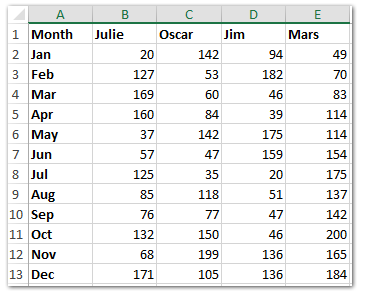이제 다음 스크린 샷과 같이 행 머리글과 열 머리글을 빈 범위 (이 예에서는 범위 A16 : E28)에 복사합니다.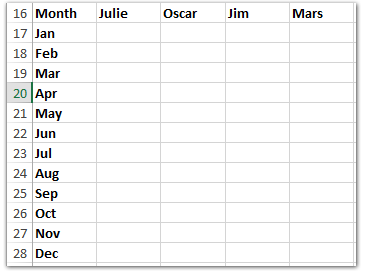Excel에서 IF 함수를 사용하는 방법

Excel에서 IF 함수의 기본 형식은 다음과 같습니다. = IF (logic_test, value_if true, value_if_false)

우리의 경우 판매량이 적거나 없는지 확인하고 싶습니다. Cell B2의 값이 80보다 작거나 같으면 "Low"텍스트를 반환하고 Cell B2의 값이 80보다 크면 공백을 반환합니다.

공식 입력 = IF (B2 <= 80, "낮음", "") 셀 B17에 넣은 다음 채우기 핸들을 B17 : E28 범위로 끕니다. 아래 스크린 샷을 참조하십시오.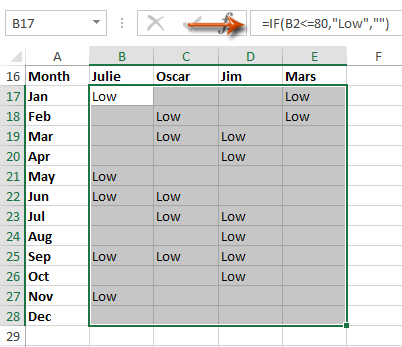여러 워크 시트 / 통합 문서 / CSV 파일을 하나의 워크 시트 / 통합 문서로 쉽게 결합

서로 다른 통합 문서의 수십 개의 시트를 하나의 시트로 결합하는 것은 지루할 수 있습니다. 그러나 Excel 용 Kutools를 사용하면 결합 (워크 시트 및 통합 문서) 유틸리티를 사용하면 몇 번의 클릭만으로 완료 할 수 있습니다! 전체 기능 무료 평가판 30일!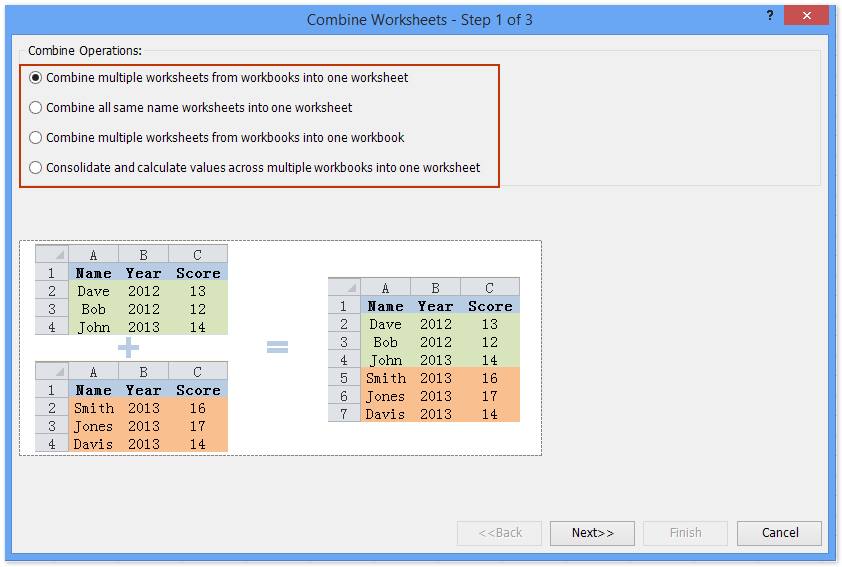Excel에서 If 함수 및 AND 함수를 사용하는 방법

셀이 동시에 여러 조건을 충족하는지 확인하려면 Excel에서 IF 함수와 AND 함수를 결합 할 수 있습니다.
Excel에서 AND 함수의 기본 형식은 다음과 같습니다. = AND (논리 1, 논리 2, 논리 3,… 등)

이제 우리의 경우 판매량이 80 개 이상 160 개 미만인지 동시에 확인하고, yes이면 "Middle"텍스트를 반환하고 no이면 공백을 반환합니다.

공식 입력 = IF (AND (B2> 80, B2 <160), "중간", "") 셀 B17에 넣은 다음 채우기 핸들을 B17 : E28 범위로 끕니다. 아래 스크린 샷을 참조하십시오.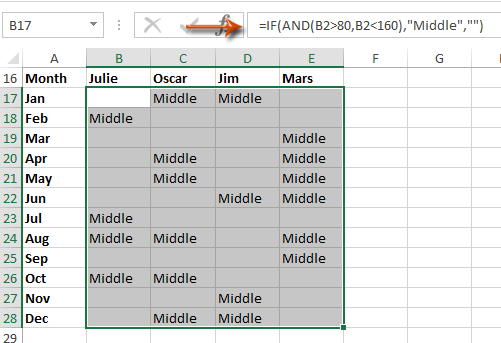Excel에서 IF 함수 및 OR 함수를 사용하는 방법

셀이 여러 조건 중 하나를 충족하는지 확인하려면 Excel에서 IF 함수와 OR 함수를 결합 할 수 있습니다.

Excel에서 OR 함수의 기본 형식은 다음과 같습니다. = OR (논리 1, 논리 2, 논리 3,… 등)

이 예에서 180보다 크거나 20보다 작은 비정상적인 판매량을 찾으려고합니다. 판매량이 비정상적인 경우 공백을 반환하지 않으면 "Unordinary"라는 텍스트를 반환합니다.

공식 입력 = IF (OR (B2> 180, B2 <20), "비정상", "") 셀 B17에 넣은 다음 채우기 핸들을 B17 : E28 범위로 끕니다. 아래 스크린 샷을 참조하십시오.여러 IF 함수를 Excel에서 하나의 수식으로 결합하는 방법

이 예에서 하나의 공식으로 모든 판매량을 평가하려는 경우 복잡한 IF 함수를 적용 할 수 있습니다.

판매량이 20 개 이하인 경우 "Unordinary"텍스트를 반환합니다. 판매량이 80보다 작거나 같으면 "낮음"텍스트를 반환합니다. 160보다 작거나 같으면 "Middle"텍스트를 반환하고 200보다 작거나 같으면 "High"텍스트를 반환합니다.

공식 입력 = IF (B2 <= 20, "비정상", IF (B2 <= 80, "낮음", IF (B2 <= 160, "중간", IF (B2 <= 200, "높음", ""))) ) 셀 B17에 넣은 다음 채우기 핸들을 B17 : E28 범위로 끕니다. 아래 스크린 샷을 참조하십시오.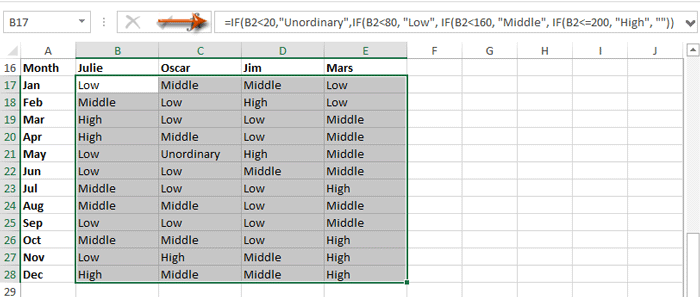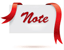수식이 너무 복잡해서 기억 나지 않습니까? 나중에 한 번만 클릭하여 재사용 할 수 있도록 수식을 자동 텍스트 항목으로 저장하십시오! 자세히보기 ...     test

최고의 사무 생산성 도구

Excel 용 Kutools는 대부분의 문제를 해결하고 생산성을 80% 향상시킵니다.

• 재사용: 빠르게 삽입 복잡한 공식, 차트 그리고 이전에 사용한 모든 것; 셀 암호화 암호로; 메일 링리스트 생성 이메일 보내기 ...
• 슈퍼 포뮬러 바 (여러 줄의 텍스트와 수식을 쉽게 편집 할 수 있습니다.) 레이아웃 읽기 (많은 수의 셀을 쉽게 읽고 편집합니다.) 필터링 된 범위에 붙여 넣기...
• 셀 / 행 / 열 병합 데이터 손실없이; 셀 내용 분할; 중복 행 / 열 결합... 중복 셀 방지; 범위 비교...
• 중복 또는 고유 선택 행; 빈 행 선택 (모든 셀이 비어 있음); 슈퍼 찾기 및 퍼지 찾기 많은 통합 문서에서; 무작위 선택 ...
• 정확한 사본 수식 참조를 변경하지 않고 여러 셀; 참조 자동 생성 여러 시트에; 글 머리 기호 삽입, 확인란 등 ...
• 텍스트 추출, 텍스트 추가, 위치 별 제거, 공간 제거; 페이징 부분합을 만들고 인쇄합니다. 셀 내용과 주석 간 변환...
• 슈퍼 필터 (다른 시트에 필터 구성표 저장 및 적용) 고급 정렬 월 / 주 / 일, 빈도 등 특수 필터 굵은 기울임 꼴로 ...
• 통합 문서와 워크 시트 결합; 키 열을 기반으로 테이블 병합; 데이터를 여러 시트로 분할; xls, xlsx 및 PDF 일괄 변환...
• 300개 이상의 강력한 기능. Office / Excel 2007-2019 및 365를 지원합니다. 모든 언어를 지원합니다. 기업 또는 조직에 쉽게 배포 할 수 있습니다. 전체 기능 30 일 무료 평가판. 60 일 환불 보장.Office Tab은 Office에 탭 인터페이스를 제공하여 작업을 훨씬 쉽게 만듭니다.

• Word, Excel, PowerPoint에서 탭 편집 및 읽기 사용, Publisher, Access, Visio 및 Project.
• 새 창이 아닌 동일한 창의 새 탭에서 여러 문서를 열고 만듭니다.
• 생산성이 50% 증가하고 매일 수백 번의 마우스 클릭이 줄어듭니다!Say something here...
symbols left.
or post as a guest, but your post won't be published automatically.
• To post as a guest, your comment is unpublished.
· 5 days ago
=IF(D4<=30000, and =>20000,than D4a-5000,and if(d4<=40000, and >30000, than d4-6000) convert into formula
• To post as a guest, your comment is unpublished.
· 2 days ago
Try the formula. Since I don't quite understand you, please check and change the part "DA4-5000" and "D4-6000", "FALSE" to the results you want.

=IF(AND(D4<=30000,D4>20000),"DA4-5000",IF(AND(D4<=40000,D4>30000),"D4-6000","FALSE"))

Amanda
• To post as a guest, your comment is unpublished.
· 1 months ago
HI EVERY ONE I NEED HELP IN THIS SYNTEX
=IF(AND(AJ=1250,AJ*2.5%),IF(AND(AJ>1250,AJ<=2500),AJ*10%,IF(AND(AJ>2500,AJ<=3750),AJ*15%,IF(AND(AJ>3750,AJ<=11666),AJ*20%))))
THIS FOURMAIL GIVE ME #NAME
WHERE THE EROO
• To post as a guest, your comment is unpublished.
· 1 months ago
Hi ahmed.dba,

Can you send the file to amanda@extendoffice.com? And if you have private information in the file, please delete them.

Amanda
• To post as a guest, your comment is unpublished.
· 1 months ago
I am trying to write a formula to tell me if I am available or busy on specific days and dates (I am always busy on certain days of the week, but then also on specific dates).
What I have so far is:
=IF(OR(A226="Monday",A226="Wednesday",A226="Thursday",A226="Friday",\$Z\$226:\$Z\$255=B226),"Busy","Available")
Columns A is the day of the week as text, column B is the day of the month as a number,
• To post as a guest, your comment is unpublished.
· 1 months ago
Hi Andrea,

Can you send the file to amanda@extendoffice.com? And if you have private information in the file, please delete them.

Amanda
• To post as a guest, your comment is unpublished.
· 1 years ago
I need help to make a formula
Quantity Count 1 Count 2 Count 3
10837 10874 10874 0
If count =0, make count 2-Quantity, if is <> 0, make count 3-Quantity, and if count2 and count 3 =0, make count 1-Quantity

• To post as a guest, your comment is unpublished.
· 2 years ago
I need help combining these IF formula:
IF D7<>C\$1 "OOP", "Current"
IF D7=C\$1 and S7=0, T7>0, "No Rev"
IF D7=C\$1 and S7<0, T7> 0, "Loss"
IF D7=C\$1 and S7<0, R=0, "No Cost"
IF D7=C\$1 and X7<=30%, "High GP"
IF D7-C\$1 and X7>=5%, "Low GP"
• To post as a guest, your comment is unpublished.
· 2 years ago

=IF fr2=31 (FT4>30,18000,FR4*FQ4) and IF fr2=30 (FT4>29,18000,FR4*FQ4)

i want to combine this.

• To post as a guest, your comment is unpublished.
· 2 years ago
Hi there, I need this to return the value in the column B6:B28, when either an H or an S are in the boxes I6:I28, works fine without the OR and just H, but not with the OR function, can you possibly help?=IF(AF8=I5,TEXTJOIN(",",FALSE,IF(OR(I6:I28="H",I6:I28="S"),B6:B28,"")))

Many thanks

David
• To post as a guest, your comment is unpublished.
· 2 years ago

If A1 begins with 2 zeros (00) return the number 1, if not return the number 2
• To post as a guest, your comment is unpublished.
· 2 years ago
8. To be a Bunk Leader, a staff member must have over 4 service years and have completed leadership training.
In cell L2, enter a formula using the IF and AND functions, as well as structured references to determine if Adam Moriarty can be a bunk leader.
a. The logical test in the IF function should determine if the staff member’s Service Years is greater than 4 AND the staff member’s Leadership Training status is “Yes”. Remember to use a structured reference to the Service Years and the Leadership Training columns.
b. The function should return the text Yes if a staff member meets both of those criteria.
c. The function should return the text No if a staff member meets none or only one of those criteria.
• To post as a guest, your comment is unpublished.
· 2 years ago
Hi Alice,
Supposing the number of service year is placed in Cell B2, the leadership training stats is placed in Cell C2, you can use this formula =IF(AND(B2>4,C2="Yes"),"Yes","No")
• To post as a guest, your comment is unpublished.
· 3 years ago
hello!
I need your brilliant minds to resolve a problem:
if a1=blank & b1=blank & c1=blank & d1=blank then result1
if a1=blank & b1=blank & c1=blank & d1>0 then result2
if a1=blank & b1=blank & c1>0 & d1>0 then result3
if a1=blank & b1>0 & c1>0 & d1>0 then result4
if a1>0 & b1>0 & c1>0 & d1>0 then result5
i've tried with if/and, if/or but it seams i can't get a result.

• To post as a guest, your comment is unpublished.
· 2 years ago
=IF(AND(ISBLANK(A1);ISBLANK(B1);ISBLANK(C1);ISBLANK(D1));1;IF(AND(ISBLANK(A1);ISBLANK(B1);ISBLANK(C1);D1>0);2;IF(AND(ISBLANK(A1);ISBLANK(B1);C1>0;D1>0);3;IF(AND(ISBLANK(A1);B1>0;C1>0;D1>0);4;5))))
• To post as a guest, your comment is unpublished.
· 3 years ago
Need some help with logic. I wanted to check the first cell for data if it has data then it will return that cell; if it doesn't have data then it will return a different cell. But if the second cell is blank then it should return a msg. Can you please help.
• To post as a guest, your comment is unpublished.
· 3 years ago
Hi Ahmed,
Supposing the first data in Cell A1, and the second data in Cell A2, you can apply the formula as =IF(A1="",IF(A2="","NO DATA", A2),A1)
• To post as a guest, your comment is unpublished.
· 3 years ago
IF(D15>30,"Organic Soils",IF(AND(D15>25,D15<29.99),"Clayey Soil (fine)",IF(AND(D15>20,D15<24.99),"Loamy Soil (moderately fine)",IF(AND(D15>10,D15<19.99),"Loamy Soil (medium)",IF(AND(D15>5,D15<9.99),"Loamy Soil (moderately coarse)",IF(D15<4.99,"Sandy Soil (coarse)")))))) Can you find the error?
• To post as a guest, your comment is unpublished.
· 3 years ago
A,b,c,d,e,f,g,a,y
• To post as a guest, your comment is unpublished.
· 3 years ago
Name Amount Profit Range
A 10,000
B 50,000
C 42,500
D 75,000
E 85,000
F 23,500
G 16,000
I 57,000
J 81,000

Profit Range Name
Less than 10,000 A
10,000-20,000 B
20,000-30,000 C
30,0000-50,000 D
50,0000-75,000 E
75,000-1,00,000 F

Write profit range in 1st table.
any Please say how to write formula for this
• To post as a guest, your comment is unpublished.
· 3 years ago
i required how to steel calculation as per dia (8,10,12,16,20,25,32) and cutting length no of bars and numbers etc

wise in excel format
• To post as a guest, your comment is unpublished.
· 3 years ago
how to use if condition for condition like if value of x lying in 60-80
• To post as a guest, your comment is unpublished.
· 4 years ago
Hello all,

I am trying to build an IF statement to help with our Service Level calculations. We need one Queue Name to have a different time requirement than all of the other queues. I am trying to figure out how to get the formula to count all Queue Names (Column B) to show they are within our Service Level Agreement (SLA) if they are in the queue for less than 30 seconds. However, for the Queue named TS_DX_CP_ES*, I need it to show it is within SLA if less than 90 seconds.

I currently have the following formula in place and it works for the 90 second rule but if I try to next the IF formula and change the Queue Name and Time Requirement, I get an error.

Formula that works currently =IF(AND([@[Queue Name]]="TS_DX_CP_ES*",[@[Queue time]]<0.00104166666666667),1,0)

Formula that gives a #VALUE! Error - =IF(AND([@[Queue Name]]="TS_DX_CP_ES*",[@[Queue time]]<0.00104166666666667),1,0),IF(AND([@[Queue Name]]="TS_PLU_US*",[@[Queue time]]<0.000358796296296296),1,0)
• To post as a guest, your comment is unpublished.
· 4 years ago
Hello all,

I am trying to build an IF statement to help with our Service Level calculations. We need one Queue Name to have a different time requirement than all of the other queues. I am trying to figure out how to get the formula to count all Queue Names (Column B) to show they are within our Service Level Agreement (SLA) if they are in the queue for less than 30 seconds. However, for the Queue named TS_DX_CP_ES*, I need it to show it is within SLA if less than 90 seconds.

I currently have the following formula in place and it works for the 90 second rule but if I try to next the IF formula and change the Queue Name and Time Requirement, I get an error.

Formula that works currently =IF(AND([@[Queue Name]]="TS_DX_CP_ES*",[@[Queue time]]<0.00104166666666667),1,0)

Formula that gives a #VALUE! Error - =IF(AND([@[Queue Name]]="TS_DX_CP_ES*",[@[Queue time]]<0.00104166666666667),1,0),IF(AND([@[Queue Name]]="TS_PLU_US*",[@[Queue time]]<0.000358796296296296),1,0)

• To post as a guest, your comment is unpublished.
· 4 years ago
Hi,

In a row, 3 columns with different value must show a different interpretation in the target cell.

=IF(A3>0,"Welcome",IF(B3>0,"Win",IF(C3>0,"Will","HI")))

Thanks Pinaki
• To post as a guest, your comment is unpublished.
· 4 years ago
Hi Pinaki,
In your formula, If A3 > 0, both B3 and C3 also might be greater than 0. Similarly, when B3 > 0, both A3 and C3 also might be greater than 0.

Normally, in the If function, you can set =IF(A3>0, "Welcome",””), which means when A3>0, returns “welcome”, while when A3 is not less than 0, returns nothing. In this function, the two condition A3>0 and A3<=0 are exclusive.

In my example =IF(B2<=20,"Unordinary",IF(B2<=80, "Low", IF(B2<=160, "Middle", IF(B2<=200, "High", "")))), The third condition B3<=160 includes the second condition B2<=80, and the second contains the first too.
• To post as a guest, your comment is unpublished.
· 4 years ago
Column A has Numerical Values. Column C & D have text.

If 2 or more separate Numerical Values in any row of Column A match then evaluate those Rows in Column C & D for any Text that doesn't match a given Text and count as 1. If Text does match then a value of 0 is assigned.

So if..

A1:A2 = 1

A3:A4 = 2

C1=Dog
C2=Cat
C3=Dog
C4: Horse

D1=Cat
D2=Dog
D3=Horse
D4=Dog

Since A1 & A2 match each other, evaluate C1,C2,D1,D2 and if any cells do not match either "Dog" or "Cat" assign a value of 1. In this example, since all cells contain either "Dog" or "Cat" a value of 0 would be assigned.

Furthermore,

Since A3 & A4 match each other, evaluate C3,C4,D3,D4 and if any cells do not match either "Dog" or "Cat" assign a value of 1. In this example, since cells C4 & D3 contain something other than "Dog" or "Cat" a value of 1 would be assigned.

• To post as a guest, your comment is unpublished.
· 4 years ago
Hi Cab,
To know more about the IF function, I guess this webpage is helpful.
https://support.office.com/en-us/article/IF-function-69aed7c9-4e8a-4755-a9bc-aa8bbff73be2
• To post as a guest, your comment is unpublished.
· 4 years ago
Hello,
What would the equation for the following be?

In cell K2 enter a formula using the IF and OR functions, as well as structured references, to determine if Adam Moriarty can be a group leader.
a. The IF function should first determine if the staff member’s Service Years is greater than 3 OR if the staff member’s college graduate status is “Yes”. Remember to use a structured reference to the Service Years and the College Graduate columns.
b. The function should return the text Yes if a staff member meets one or both of those criteria.
c. The function should return the text No if a staff member meets neither of those criteria.
• To post as a guest, your comment is unpublished.
· 4 years ago
Hi jessjess,
Thank you for your comment. I am sorry I can’t figure out a proper formula to meeting your conditions. To know more about the IF function, I guess this webpage is helpful.
https://support.office.com/en-us/article/IF-function-69aed7c9-4e8a-4755-a9bc-aa8bbff73be2
• To post as a guest, your comment is unpublished.
· 4 years ago
• To post as a guest, your comment is unpublished.
· 4 years ago
Hi Haris,
• To post as a guest, your comment is unpublished.
· 4 years ago
III
II
II
II

II
II
II
II

How can I make a formula in excel -If any one value of the column is III the result shall be displayed as III, if any value of the column is II then the result shall be displayed as II if II and III not present it shall be displayed as I
• To post as a guest, your comment is unpublished.
· 4 years ago
How would I write this into one function?

If D26 and E26 are blank, then C26-L7
If D26 is not blank and E26 is, then D26-L7
If E26 is not blank, then E26-L7
• To post as a guest, your comment is unpublished.
· 4 years ago
=IF(AND(D26="",E26=""),(C26-L7),IF(AND(D26<>"",E26=""),D26-L7,E26-L7))
• To post as a guest, your comment is unpublished.
· 4 years ago
I am trying to write a formula to look at

1. Is the figure either over £1000, or under -£1000

2. Plus is the next cell over 55% or more

so far I have =OR(M4>=1000,M4<=-1000) which pulls in the over or under £1000 plus or minus but am unsure how to add the second criteria presume its an AND(N2>=55%) but when I add it to the formula it doesnt work.
• To post as a guest, your comment is unpublished.
· 4 years ago
Hi Michelle,
• To post as a guest, your comment is unpublished.
· 4 years ago
Hi. How can I write this as formula?

If year is equal to current year, add 1.
if year is previous year, add 1 OR start 1.
if year is 2 years prior, add 1 OR start 1.
• To post as a guest, your comment is unpublished.
· 4 years ago
Hi Everyone, I need to write a formula for the following:

IF A1 = "BOTTOMS" AND A9 <14, then 14, otherwise A9

OR A1 = "TOPS" AND A9 <5, then 5, otherwise A9
• To post as a guest, your comment is unpublished.
· 4 years ago
Hi FG，
You can’t create a formula to meeting one of both your conditions in Excel.
• To post as a guest, your comment is unpublished.
· 4 years ago
Hi Kelly!

I actually figured out a workaround!

=ROUND((IFERROR((IF(OR(AND(\$I9="BOTTOMS",BL9>=\$BY\$3),AND(\$I9="TOPS",BL9>=\$BY\$4)),BL9,VLOOKUP(\$I9,\$BX\$3:\$BY\$4,2,FALSE))),0)),0)

I9 - populates "BOTTOMS" or "TOPS" dependant on merchandise code entered
BL9 - minimum set defined by store indice
BY9 - minimum set defined by buyer (i.e the 14 units for bottom and and the 5 units for tops)
BX\$3:\$BY\$4 - vlookup to minimum set defined by buyer if if statement is false
• To post as a guest, your comment is unpublished.
· 4 years ago
Hello! I am currently creating a template that will provide me a value depending on the score of the trainee.

I was hoping I can add a value of 5 for any team member who gets a certain quiz %

Here's what I'm thinking.

5= 100%
4= 99.9% to 90%
3= 90% to 85%
2= 85% to 80%
1= <79.99

I hope you guys can help me with the formula
• To post as a guest, your comment is unpublished.
· 4 years ago
Hello, I have a pretty complex formula I am trying to write and it is killing me to admit defeat on this one. So far I have:

=IF(AND(B2="Prudential",OR(C2={"10 Year Term","15 Year Term","20 Year Term","30 Year Term"})),D2*0.75,(IF(C2="UL",D2*0.8,"0")))

and it works just fine. However, I need to add about 37 more companies with their own commission structures to it. I am pretty sure I just need one monster OR statement with a bunch of nested IFs thrown in I just am at my wits end on figuring out exactly where to place them. Any help would be amazing. Thank you!
• To post as a guest, your comment is unpublished.
· 4 years ago
Hi Dan,
Your question is still unclear. you might to explain more by providing a solution for at least 2 companies. However, just help to shorten your OR function a bit. OR(C2={"10","15","20","30"}&" Year Term").
• To post as a guest, your comment is unpublished.
· 4 years ago
=IF(OR((I2=(T2+U2),(I2=(U2+V2)),"Closed","OPEN")
HOW TO MAKE THIS RIGHT?
• To post as a guest, your comment is unpublished.
· 4 years ago
=IF(OR(I2=T2+U2,I2=U2+V2),"CLOSED","OPEN")
• To post as a guest, your comment is unpublished.
· 4 years ago
Im trying to do IF(OR(A1=1;AND(A2=1;A3=1)))
• To post as a guest, your comment is unpublished.
· 4 years ago
Hi Mirko,
Thank you for your comment. What calculation result do you want to get? Could you tell me more about your problem?
• To post as a guest, your comment is unpublished.
· 4 years ago
I'm trying to make the formula return 4 possible answer which is (R-regular,A-absent,L-late,OT-overtime). My basis for regular is if the value of the cell is "8". So this is the formula that I made so far =IF(H16>7.9,"OT",IF(H16<7.9,"L",IF(H16=8,"R","A"))). It only returns (OT and L) something is very wrong and i can't figure it out. I'm just new to excel so please help me
• To post as a guest, your comment is unpublished.
· 4 years ago
Hi Dan,
Try this formula =IF(H16=8,"R",IF(H16<7.9,"OT","L"))
• To post as a guest, your comment is unpublished.
· 4 years ago
I'm trying to make the formula return 4 possible answer which is (R-regular,A-absent,L-late,OT-overtime). My basis for regular is if the value of the cell is "8". So this is the formula that I made so far =IF(H16>7.9,"OT",IF(H16<7.9,"L",IF(H16=8,"R","A"))). It only returns (OT and L) something is very wrong and i can't figure it out. I'm just new to excel so please help me.
• To post as a guest, your comment is unpublished.
· 4 years ago
Try this: =IF(OR(H16=0,H16=""),"A",IF(H16=8,"R",IF(H16<7.9,"L","OT")))
• To post as a guest, your comment is unpublished.
· 4 years ago
how to determine the age of the cell ? is this formula right =IF(I2<30,"future payment",AND(IF(I2>30<=45,"30-45days",AND(IF(I2>45<=60,"45-60days",AND(IF(I2>60,"61+days
",0)))))))
• To post as a guest, your comment is unpublished.
· 4 years ago
Hi pramod,
You cannot use I2>30<=45 in formula directly. I have changed the formula for you: =IF(I2<=30,"FUTURE PAYMENT",IF(B2<=45, "30-45 DAYS", IF(B2<=45, "45-60DAYS", IF(B2<=60, "60+DAYS", ""))))
• To post as a guest, your comment is unpublished.
· 4 years ago
I have charges calculation for eg. charges amount is 0 then next column should be 0. if charges is more than 1 and less than 250 then next column should be 250. If charges is more than 250 then actual charges should come in next column(252)
• To post as a guest, your comment is unpublished.
· 5 years ago
how do i make this formulas talk to one another:

=IF(OR(AND(MID(K2,6,1)="N",(MID(K2,6,1)="C"),(MID(K2,6,1)="H"),(MID(K2,6,1)="I"),(MID(K2,6,1)="B"),(MID(K2,6,1)="F"),(MID(K2,6,1)="L"),(MID(K2,6,1)="M"),(MID(K2,6,1)="P"),(MID(K2,6,1)="R"),(MID(K2,6,1)="P"),ISTEXT(G2)="61"),OR(AND(RIGHT(K2,2)=G2))),"Good","Review")

=IF(AND(RIGHT(K2,2)=G2),"Good","Review")

or =IF(NOT(OR(AND(MID(K2,6,1)="N",(MID(K2,6,1)="C"),(MID(K2,6,1)="H"),(MID(K2,6,1)="I"),(MID(K2,6,1)="B"),(MID(K2,6,1)="F"),(MID(K2,6,1)="L"),(MID(K2,6,1)="M"),(MID(K2,6,1)="P"),(MID(K2,6,1)="R"),(MID(K2,6,1)="P"),ISTEXT(G2)="61"),(RIGHT(K2,2)=G2))),"Good","Review")